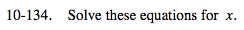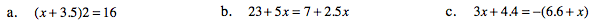### Home > MC2 > Chapter 10 > Lesson 10.2.5 > Problem10-134

10-134.Begin by distributing 2 to the terms inside the parentheses.

x = 4.5

Move all variables to one side of the equation and all constants to the other side of the equation.

Remember to distribute the negative inside the parentheses.
3x + 4.4 = −6.6 − x

Add x to both sides and subtract 4.4 from both sides to isolate x.
4x = −11

x = −2.75